## Software Development-Role of Automation in analysis phase

On 05:49 by Vardan Kumar in , ,    No comments
Automation-Analysis

## Software Development-Role of Automation in analysis phase

What is Automation and why it should be performed?

Well, automation is any technique introduced to let our machine perform some task without any manual intervention or some manual intervention can be used if it is semi-automation. Machine can perform the task through a script , a code or an application depending on the requirement.

For Example

Writing a code that would perform repetitive database operations as in suppose inserting a large amount of data in database through an excel workbook. This kind of task can be so tedious when trying to do manually.

Why it should be performed?

• In today's world time is money, you got it right definitely to save some of  those intense man hours, an automation process can be introduced.
• Learning is the key: Well while in analysis phase of a software development life cycle, a lot of documentation is required, the data capture if done manually could not add much your skill set, but writing some script or code to automate the documentation process would add a lot to your skills.
• Make your work interesting: Imagine, what if you spend your working hours capturing data in excel workbook, well sounds really boring, right! Letting your code do the same would definitely save those boring hours.
When Automation should not be done?

It is not always advised to automate the work, Whenever your work is not repetitive and building some code or script may take more time than your work, don't go for automation off course.

Analysis Phase in Software development Life cycle:

Analysis is one of the most important phase in any software development life cycle. A lot of documentation is required in this phase. Analysis could be any as in impact analysis on source files for a particular change orders, fetching dependencies or selecting some data from database as in describing tables etc. .

Instead of spending lot of hours digging your eyes into the computer screen it is better to write a code once according to your requirement and let your code do the rest.

Doing this will ensure accuracy, if all scenarios are taken care of off course, add a lot to your logic building skills as well as skill set and also if you are a part of a major project you can share your application with your team mates. This will eventually get you more rating bands off course.

Let us discuss some scenarios where automation can play a crucial role in the analysis phase of the software development life cycle....Automation Analysis

Scenarios

• Suppose you are a part of a major project in which a certain identifier's name is required to be changed and you or your team are required to prepare an impact analysis document where you have to capture the source files or the part of project's code being impacted with that identifier and main thing you have thousands of source files that are need to be traversed. Now what would you do
• Either spend those boring hours, opening your source files and count the number of occurrences of that identifier if any and capture it an excel workbook.
• Or write a code once and let your machine generate impact analysis document for you.
• Code could be any, a small c# windows form application would do or a small shell script can serve the purpose
• Let us consider another scenario, suppose you have a list of  hundreds or thousands of database tables for which you have to capture number of records corresponding to a table along with all its column alongside their data types in an excel workbook, what would you do.
• Either connect to database, describe each and every table, capture columns alongside its datatype for the same and select count(*) from table_name.
• Or provide list of tables in a flat file and let your code process on each and every table one by one and capture the required data for you in an excel workbook for the same.
• Use of system tables in a small c# application would solve the purpose.
• Let us consider one more scenario, suppose you are to capture relative path of a file in which a particular identifier is being referred to in your your local directory or a sub-directory.
• Now doing it manually capturing data or path could be tedious and kind of a good for nothing task.
• Building an application that would capture path along side file name for you sounds quite interesting and a productive task.

There are a lot more scenarios that no one can imagine of. Doing such automation could be productive upto a great extent, its just the matter of choice.

## How to get all the file names along with number of hits of a particular word under a directory or sub-directory in c#

On 00:20 by Vardan Kumar in    No comments
No. of Hits in C#

## How to get all the file names which contains a particular word

Analysis is the most important step which every software development life cycle requires to avoid rework. Sometimes it becomes a tedious task and could eat up a lot of time hence increasing cost of software development. For example: Some code changes in the current system needs to be implemented, changes like you have to change a particular identifier name to another in the entire system. So if this process is needed to be carried out professionally then the impact analysis of that identifier should be captured. So what would you open each and every source file, header files or what ever your system contains and try to capture, how many times the identifier hits in a particular file, well reading thousands of files having thousands of line definitely not feasible. So instead of manually doing this task, automation should be implemented for the same.

So let us make c# windows form application which will take directory as well as identifier name from the user and returns a txt file or excel file or what ever format you want and get all the file names along with the number of hits of a word in a particular file.

### Logic

• Let the user enter the identifier name and select the directory.
• Validations should be applied i.e if user leaves the field blank or do not select a directory.
•  if (String.IsNullOrEmpty(textBox1.Text))
{
return;
}
• We'll use a list<string> to add as one complete row to data table which in turn will become one row of excel.
• The contents of <list string> will be in sequence, first the name of file and second the no. of hits corresponding to that file.
• The list will be added as row to data table.
• List will be cleared so as to populate a row for next file in data table.
• Add columns to data table giving your desired names. Also check if data table already contains columns, what if a user in a single run search and retrieve more than once.
•  if(dt.Columns.Count==0)
{
dt.Columns.Add("Total No. of Hits for SAK");
}
• Now for each file in the selected directory enabling search option to search all directories and specifying desired file format(* to search all file formats).
• foreach (string file in Directory.EnumerateFiles(dir, "*", SearchOption.AllDirectories).
• Copy all the contents of the file at once in a string.
• Check if the entered identifier name exist in that file.
•  if (contents.IndexOf(textBox1.Text, StringComparison.OrdinalIgnoreCase) >= 0
• Use string extension to ignore case.
•  public static class StringExtensions
{
public static bool Contains(this string source, string toCheck, StringComparison comp)
{
return source.IndexOf(toCheck, comp) >= 0;
}

}
• If the file contains the identifier name then add the file name to list string.
add = String.Empty; //To clear string for next file.
• Now since we are sure that the current file contains at least an occurrence of entered identifier name we will read the contents copied to the string line by line.
{
string line;

• Now check if identifier exists in the current line, again ignoring case
• if (line.IndexOf(textBox1.Text, StringComparison.OrdinalIgnoreCase) >= 0)
• Now if identifier exists in that line, count the total number of occurrences of the identifier in that line.
• while ((pos < line.Length) && (pos = line.IndexOf(textBox1.Text,pos,StringComparison.OrdinalIgnoreCase)) != -1)
{
ofile_occur++;
pos += textBox1.Text.Length;
}
ofile_occur = 0;  // for re-use
•             pfilr_occur=pfilr_occur+ofile_occur;
• Now after reading all the lines of a particular file. pfilr_occur contains all the hits of that particular file. Just add that to list<string> and that list string as a row to data table. Don't forget to clear the list string to re-use and the file occurrence variable
dtrow.Clear();
pfilr_occur = 0;.
• Repeat the above steps for each and every file.
• Finally after traversing through all the files in all the directories as well as sub-directories as specified by the user data table dt is ready.
• Now just populate the excel sheet using that data table.
• Excel sheet is ready make it visible to use and save the excel sheet, if you want to specify a unique path for the excel file for the sake of file versioning
• Note: Folder Browser dialog is used for user to select a directory.
• private void button2_Click(object sender, EventArgs e)
{
DialogResult result = folderBrowserDialog1.ShowDialog();
if (result == DialogResult.OK)
{
dir = folderBrowserDialog1.SelectedPath;
label3.Text = dir;
tt.SetToolTip(label3, label3.Text);  // How to set tool tip in c#
}
}
• Also if the identifier name is not found then a message box should be displayed.
• else
{
MessageBox.Show("Column name not found in any of the files in specified directory");
}File name along with number of hits in c#

### Source Code

using System;
using System.Collections.Generic;
using System.ComponentModel;
using System.Data;
using System.Drawing;
using System.Linq;
using System.Text;
using System.Windows.Forms;
using System.IO;
using System.Diagnostics;
using System.Text.RegularExpressions;
using Microsoft.Office.Interop.Excel;

namespace WindowsFormsApplication3
{
public partial class Form1 : Form
{
System.Data.DataSet dataSet = null;
System.Data.DataTable dt = new System.Data.DataTable("sak_impact");
int file_count = 0;
int occur_count=0;
int total = 0;
int ofile_occur = 0;
int pfilr_occur = 0;

List<String> dtrow=new List<String>();

ToolTip tt = new ToolTip();
String dir = String.Empty;
public Form1()
{
InitializeComponent();
}

private void button1_Click(object sender, EventArgs e)
{
int emflag = 0;
if (String.IsNullOrEmpty(textBox1.Text))
{
return;
}

if (String.IsNullOrEmpty(dir))
{
return;
}
if(dt.Columns.Count==0)
{
dt.Columns.Add("Total No. of Hits for SAK");
}
foreach (string file in Directory.EnumerateFiles(dir, "*", SearchOption.AllDirectories))
{
if (contents.IndexOf(textBox1.Text, StringComparison.OrdinalIgnoreCase) >= 0)
{
emflag = 1;
{
string line;
{
if (line.IndexOf(textBox1.Text, StringComparison.OrdinalIgnoreCase) >= 0)
{

int pos=0;
while ((pos < line.Length) && (pos = line.IndexOf(textBox1.Text,pos,StringComparison.OrdinalIgnoreCase)) != -1)
{
ofile_occur++;
pos += textBox1.Text.Length;
}
pfilr_occur=pfilr_occur+ofile_occur;
ofile_occur = 0;

}
}

}
dtrow.Clear();
pfilr_occur = 0;
}
}

if (emflag == 1)
{
dataSet = new DataSet("General");
Microsoft.Office.Interop.Excel.Application ExcelApp = new Microsoft.Office.Interop.Excel.Application();

// Loop over DataTables in DataSet.
DataTableCollection collection = dataSet.Tables;

Sheets xlSheets = null;
Worksheet xlWorksheet = null;
//Create Excel Sheets
xlSheets = ExcelApp.Sheets;
Type.Missing, Type.Missing, Type.Missing);

System.Data.DataTable table = collection;
xlWorksheet.Name = table.TableName;
for (int j = 1; j < table.Columns.Count + 1; j++)
{
ExcelApp.Cells[1, j] = table.Columns[j - 1].ColumnName;
}

// Storing Each row and column value to excel sheet
for (int k = 0; k < table.Rows.Count; k++)
{
for (int l = 0; l < table.Columns.Count; l++)
{
ExcelApp.Cells[k + 2, l + 1] =
table.Rows[k].ItemArray[l].ToString();

}
}
ExcelApp.Application.ActiveWindow.WindowState = Microsoft.Office.Interop.Excel.XlWindowState.xlMaximized;
ExcelApp.Application.ActiveWindow.FreezePanes = false;
ExcelApp.Application.ActiveWindow.SplitColumn = 1;
ExcelApp.Application.ActiveWindow.SplitRow = 1;
ExcelApp.Application.ActiveWindow.FreezePanes = true;
ExcelApp.Columns.AutoFit();
//  xlWorkbook.SaveAs("");
ExcelApp.Visible = true;
((Worksheet)ExcelApp.ActiveWorkbook.Sheets[ExcelApp.ActiveWorkbook.Sheets.Count]).Delete();

}
else
{
MessageBox.Show("Column name not found in any of the files in specified directory");
}
}

private void label2_Click(object sender, EventArgs e)
{

}

private void button2_Click(object sender, EventArgs e)
{
DialogResult result = folderBrowserDialog1.ShowDialog();
if (result == DialogResult.OK)
{
dir = folderBrowserDialog1.SelectedPath;
label3.Text = dir;
tt.SetToolTip(label3, label3.Text);
}
}

}
public static class StringExtensions
{
public static bool Contains(this string source, string toCheck, StringComparison comp)
{
return source.IndexOf(toCheck, comp) >= 0;
}

}
}

## Troubleshooting:Unable to set the Freeze Panes property of Window Class C# with reason or How to freeze panes of excel in C#

On 06:01 by Vardan Kumar in ,    No comments

## Unable to set the Freeze Panes property of Window Class C#

It is generally easy to resolve the compile time errors because the reason for the error is probably known but a run time error always hinders the development process for a considerable amount of time so are the exceptions that unknowingly show up from no where. That is why it is always advised to handle each and every exception your application might throw. The only way to be a master in handling exception is experience, yeah you read it right. More you develop, more problem you will encounter in your developing environment and in turn more you will know the reason and the so called timing of the exceptions.

Exception

One of the most encountered is "unable to set freeze panes property of window class"  which show up when you try to freeze panes while formatting an excel file in c#.

Reason

The main reason for occurrence of such an exception is incompatibility between older and the newer versions of MS Excel.

When it occirs

This exception occurs when you are using a newer version of MS Excel and your application gets activate in background, I repeat your MS Excel application thread opens in the minimized state which hinders the freezing pane process.

How to counter this exception?

Obviously to get a solution to a problem, first of all understanding the problem is must more precisely the reason of problem should be known. Since we know the reason why freeze panes property is not set and the exception is thrown we can provide a solution.

So if somehow we can make our MS Excel thread open in maximized state or normal state explicitly then bingo we successfully resolved the issue.

Still it is a good practice to surround the exception causing snippet with try block and catch the exception so that there is no exceptional flow change in your application.Unable to Set Freeze Panes Exception

Let us look at the source code for the same

### Source Code

//Maximize the window state before setting freeze panes property

ExcelApp.Application.ActiveWindow.WindowState = Microsoft.Office.Interop.Excel.XlWindowState.xlMinimized;
ExcelApp.Columns.AutoFit();
try
{
ExcelApp.Application.ActiveWindow.FreezePanes = false;
ExcelApp.Application.ActiveWindow.SplitColumn = 1; //To freeze first column
ExcelApp.Application.ActiveWindow.SplitRow = 1; // To freeze Top Row
ExcelApp.Application.ActiveWindow.FreezePanes = true;
}

catch(Exception ex)
{

}

Freeze Panes Exception

## How to use file versioning, saving file with unique file name in c#

On 21:22 by Vardan Kumar in    No comments
File Versioning C#

## File versioning, saving file with unique file name in c#

File versioning allows a user to have several versions of a file simultaneously at a particular path. Not only it intimates a user of which is the latest version i.e generated at a later stage or an older version but also provides a unique file name in the same directory.

For example....

Suppose a report generation application is developed which saves a user-readable file as an output, as discussed in earlier posts also an efficient GUI(Graphical user interface) is the most interactive one i.e. a GUI in which a user feels to have control over the application. In such an application a user is generally provided with the privilege to select his/her desired path. It is a general tendency of a computer operating individual to select same path as well as name each time he/she wants to generate the report. Now suppose user chooses a file name as cspassion.xlsx and the same file name already exists at that path, so an efficient application would save the latest version of report as cspassion(1).xlsx or cspassion(2).xlsx to avoid exception or prompting user to select if he/she wants to replace the previous version of file or not, also this would take the control from your application to the operating system which should generally be avoided as much as possible.

### Logic

• First of all user selected file name or path is sent as an argument to the method fetching unique file path or creating file versions.
• Now we will check if the file already exists at that directory or not
• If not then return that file path and save the file with user selected file name at his/her desired path.
• If yes then continue with the version generation.
• Now split and store directory,file name as well as extension in different identifiers.
• Initialize number as 1,if you want to display (2) as file version after first version and initialize to 0 if you want it as (1) after first version.
• Now we'll check if a version after initial version is generated using regular expression match
• If it matches then we'll extract file name from group 1 and number from group 2.
• Now we have number as well as file name we'll loop through while the file exists in that path.
• We'll increment number by 1 in each iteration
• Format the string as filename,(number) and extension.
• Return the file path and save the workbook.
I have generated five versions of same file named cspassion for reference in current directory. Refer to image below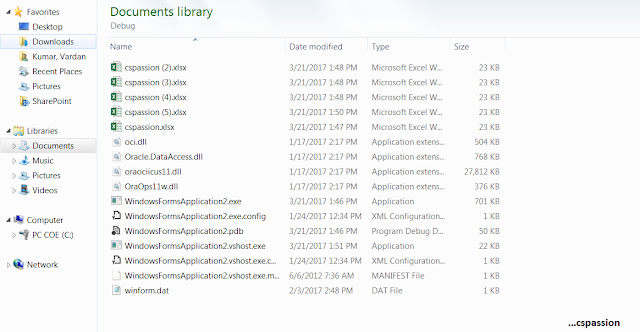File Versioning C#

### Source Code

using System.ComponentModel;
using System.Data;
using System.Drawing;
using System.Linq;
using System.Text;
using System.Windows.Forms;
using Microsoft.Office.Interop.Excel;
using Oracle.DataAccess.Client;
using System.Configuration;
using System.Text.RegularExpressions;
using System.Xml;
using System.IO;

namespace WindowsFormsApplication2
{
public partial class Form1 : Form
{
string s_oRcConnectionString = null; //Click here to know how to set dynamic connection string c#
ToolTip tt = new ToolTip();
string uniquepath=String.Empty;
private void btn_Save(object sender, EventArgs e)
{
xlWorkbook.SaveAs(uniquepath);
}
private void btn_Browse_Click_1(object sender, EventArgs e)
{
SaveFileDialog saveFileDialog1 = new SaveFileDialog();// using save file dialog
saveFileDialog1.Filter = "Excel|*.xlsx";
saveFileDialog1.Title = "Save Excel report";
if (saveFileDialog1.ShowDialog() == DialogResult.OK)
lbl_Path.Text = saveFileDialog1.FileName;
lbl_Path.Visible = true;
string path=lbl_Path.Text;
uniquepath=GetUniqueFilePath(path);

}

public static string GetUniqueFilePath(string filepath)
{
if (File.Exists(filepath))
{
string fold = Path.GetDirectoryName(filepath);
string filename = Path.GetFileNameWithoutExtension(filepath);
string extension = Path.GetExtension(filepath);
int number = 1;

Match regex = Regex.Match(filepath, @"(.+) \((\d+)\)\.\w+");

if (regex.Success)
{
filename = regex.Groups.Value;
number = int.Parse(regex.Groups.Value);
}

do
{
number++;
filepath = Path.Combine(folder, string.Format("{0} ({1}){2}", filename, number, extension));
}
while (File.Exists(filepath));
}

return filepath;
}
}
}

## 8-Bit Binary Adder program in C

On 23:37 by Vardan Kumar in ,    No comments

## 8-Bit Binary Addition in C

Binary number system is the system which is used by the computer, it is the key for really fast mathematical computations performed, so let us discuss the logic for 8-bit binary adder step by step.

### Prerequisites

Above two logic understandings are really important in order to make the inputs and outputs user as well as computer understandable. We'll discuss one full logic in here but it is recommended that you go for step by step explanation by checking out aove mentioned links.

### Logic

• Let the user input two operands, as we are dealing with 8-bit format so restrict the input from 0-255.
• Convert the two operands into its binary equivalent and store the results in two separate arrays. Displaying binary equivalent to user is optional but advised.
• Pass the two arrays and the result array as arguments to the function that would do the core computation of adding 8-bit binary numbers.
• Loop bit by bit till 8 bits i.e. from 0 to 7.
• Declaring initial carry bit as 0, do a^b^c and store the result bit in result array, where a is first input array, b is second input array and c is carry
• Evaluate the carry bit by performing ab+bc+ca i.e. (a&b) | (b&c) | (c&a).
• The result array is updated with the final result as the loop terminates.
• Return the carry bit 'c'.
• Check if the carry bit is 1 or 0.
• If it is 1 then print overflow since we are dealing with 8-bit addition and if carry at the end of computation is still 1 then the result would be 9 bits.
• If it is 0 then print the result as binary number and it is recommended to convert the 8 -bit result to its decimal equivalent.

### Understanding With Example

• Suppose the input numbers are 11 and 9.
• 8-bit Binary Equivalents: 11-> 00001011 and 9-> 00001001
• c=0,a=1 and b=1, performing result=a^b^c(1^1^0)=0,(X-or returns 0 if two operands are same and 1 if two operands are different,so 1^1=0,1^0=1).
• Carry bit=ab+bc+ca i.e (1&1)+(1&0)+(1&0)=1,(And(&) returns 0 if any of the two bits is 0 and Or(|) returns 1 if any of two bits is 1)
• c=1,a=1,b=0, performing result=a^b^c(1^0^1)=0
• Carry bit=ab+bc+ca i.e (1&0)|(0&1)|(1&1)=1
• c=1,a=0,b=0, performing result=a^b^c(0^0^1)=1
• Carry Bit=ab+bc+ca i.e (0&0)|(0&1)|(1&0)=0
• c=0,a=1,b=1, performing result=a^b^c(1^1^0)=0
• Carry Bit=ab+bc+ca i.e (1&1)|(1&0)|(0&1)=1
• c=1,a=0,b=0,performing a^b^c(0^0^1)=1
• carry Bit=ab+bc+ca i.e (0&0)|(0&1)|(1&0)=0
• Rest all the result bits will be 0.
• Final result array will be 00010100.
• Converting to its decimal equivalent will give 20(11+9).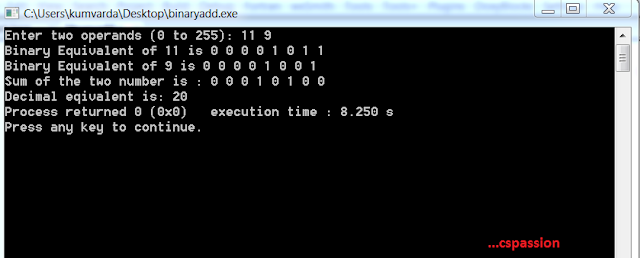8-Bit binary adder output

### Source Code

#include <stdio.h>
#include <math.h>

void decimal2Binary(int op1, int aOp[]){
int result, i = 0;
do{
result = op1 % 2;
op1 /= 2;
aOp[i] = result;
i++;
}while(op1 > 0);
}

int binary2Decimal(int array[]){
int sum = 0, i;
for(i = 0; i < 8; i++){
if(array[i]) sum += pow(2,i);
}
return sum;
}

void displayBinary(int array[], int n){
int i;
for(i = n -1; i >=0; i--){
printf("%d ", array[i]);

}
printf("\n");
}

int addBinary(int a1[], int a2[], int result[]){
int i, c = 0;
for(i = 0; i < 8 ; i++){
result[i] = ((a1[i] ^ a2[i]) ^ c); //a xor b xor c
c = ((a1[i] & a2[i]) | (a1[i] &c)) | (a2[i] & c); //ab+bc+ca
}
result[i] = c;
return c;
}

int main(){
int op1, op2, sum;
int  a = {0,0,0,0,0,0,0,0};
int  b = {0,0,0,0,0,0,0,0};
int  aSum = {0,0,0,0,0,0,0,0};
printf("Enter two operands (0 to 255): ");
scanf("%d %d", &op1, &op2);
while(op1 < 0 || op1 > 255 || op2 < 0 || op2 > 255 ){
printf("Enter two operands (0 to 255): ");
scanf("%d %d", &op1, &op2);
}
decimal2Binary(op1, a);
decimal2Binary(op2, b);
printf("Binary Equivalent of %d is ",op1);
displayBinary(a, 8);
printf("Binary Equivalent of %d is ",op2);
displayBinary(b, 8);
printf("Sum of the two number is : ");
displayBinary(aSum, 8);
sum = binary2Decimal(aSum);
printf("Decimal eqivalent is: %d", sum);
}else{
printf("Overflow,crossed 8 bits");
}
return 0;
}

## 8-Bit Binary to Decimal Conversion in C

On 21:56 by Vardan Kumar in    No comments

## Binary to Decimal Conversion

Sometimes in a program, the output is in the form of binary number system. Such output is really comfortable for a computing machine to understand but user can not understand such kind of output form i.e. binary output, hence if our program is generating a binary output a functional logic should be written in order to convert that binary to decimal number system.

### Logic

1. We'll take the input as an array or if we want to take the input directly as an integer then we have to separate the digits of that integer and store the same in an array in reverse order since we are to perform calculations bit by bit. Suppose we enter 0 0 0 0 1 1 0 1.
2. Next for every 1 in our array we'll add the previous addition with 2 to the power,position of that element in array,
3. Since we will store the values in array in reverse order hence 0th index will be 1 followed by 0 at 1st index and so on, giving our array in sequence as 1 0 1 1 0 0 0 0.
4. Now sum=1*2^0=1 in iteration.(1 at index 0)
5. Again for next 1 we will have sum=1+2^2=5.(1 at index 2)
6. Again for next 1 we will have sum=5+2^3=13(1 at index 3)
7. Since there is no 1 there after hence we have 13 as our binary equivalent for the given input.Output Binary to Decimal

Source Code

#include<Stdio.h>
#include<math.h>

int Binary2Decimal(int aBin[]){
int sum = 0, i;
for(i = 0; i < 8; i++){
if(aBin[i])
sum += pow(2,i);
}
return sum;
}

void main()
{
int iBin,i,cDec;
printf("Please enter 8 bit binary integer bit by bit\n");
for(i=7;i>=0;i--)
{
scanf("%d",&iBin[i]);
}
cDec=Binary2Decimal(iBin);
printf("The equivalent decimal is %d",cDec);
}

If n bit conversion is required use of macros is recommended in order to write a generic program for the same, logic will be same.

## 8-Bit Decimal to Binary Conversion program in C

On 02:53 by Vardan Kumar in    No comments
Decimal to Binary Conversion

## Decimal to Binary Conversion

The most common number system we normally use in ff course decimal number system i.e. numbers from (0-9) but when it comes to computing class decimal number system is not really common rather computer systems use binary number system for computing purposes whether it is digital encoding or a discrete mathematics branch called Boolean Algebra. Hence once should be aware of the logic to convert a decimal number system to its respective binary equivalent.

### Logic

We will use the theoretical approach as its programmatic implementation to fulfill our desired purpose of converting decimal number system to its binary twin. Also we'll fetch our result bit by bit so we will use an array to store the bits.

1. So first of all we'll take our operand and divide it with two.
2. We'll store the remainder as our result bit in the array.
3. And we will loop through till our operand is greater than 0.
This was the computation logic. Note in order to display the result we'll traverse the array in reverse order and print the result bit by bit. Also we can modify the number of bits to our desired number, We recommend use of macros for that purpose.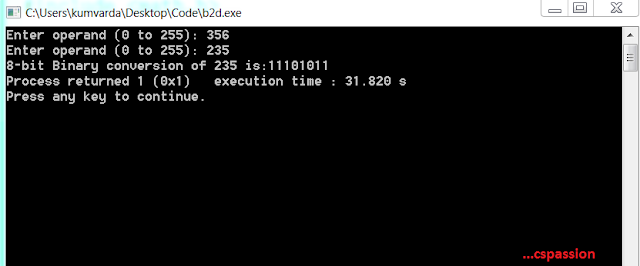Output:Decimal to Binary Conversion

### Source Code

#include <stdio.h>
#include <math.h>

void Decimal2Binary(int op, int bop[]){
int result, i = 0;
do{
result = op % 2;
op /= 2;
bop[i] = result;
i++;
}while(op > 0);
}
void main()
{

int op,i;
int  aOp = {0,0,0,0,0,0,0,0};

printf("Enter operand (0 to 255): ");
scanf("%d", &op);
while(op < 0 || op > 255)
{
printf("Enter operand (0 to 255): ");
scanf("%d", &op);
}
Decimal2Binary(op, aOp);
printf("8-bit Binary conversion of %d is:",op);
for(i=7;i>=0;i--)
{
printf("%d",aOp[i]);
}
}

Also read 8-Bit Binary to Decimal Conversion

## How to give save path through browse button c#

On 21:37 by Vardan Kumar in    No comments
Save Path C#

## How to give save path through browse button c#

For an application to be user friendly, it is necessary to provide maximum accessibility features. Since our application should follow abstraction and should focus on hiding complexities to user, so a good GUI(Graphical user interface) with accessibility features will show your hard work while building the application. No matter how much you burn midnight oil while developing back-end of your application, if it lacks a good GUI with accessibility features it is definitely in vain.

If your application generates a file, which user is supposed to read or gather information then giving privilege of choosing the save  path for the file to user is always nice. Button control is the best way to do this.

### Logic

1. Firstly we'll create a button click event.
private void btn_Browse_Click_1(object sender, EventArgs e)

2. Now we'll create an instance of SaveFileDIalog.

SaveFileDialog saveFileDialog1 = new SaveFileDialog();

3.Now we'll set the filter for our save file dialog that is what kind of file user should be prompted to save which is shown under save as type drop down. Multiple filters are separated by vertical bar.

saveFileDialog1.Filter = "Excel|*.xlsx";

4.Now we'll give title to our dialog box.

saveFileDialog1.Title = "Save Excel report";

5.Now we'll call ShowDialog method and if method return value seems correct then we'll assign the path to our desired label, off course we want to store the file path selected by user to use it further. If you don't want to display the path selected by user you can also use a string identifier to store the path.

if (saveFileDialog1.ShowDialog() == DialogResult.OK)
lbl_Path.Text = saveFileDialog1.FileName;

Note: Don't forget to change other characteristics of label if any after changing its text such as tool tip etc.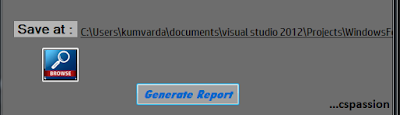Path Before clicking browse buttonSave File Dialog Box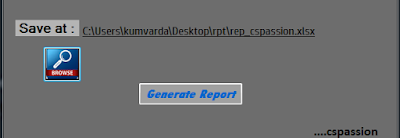Save Path after selecting path

### Source Code

private void btn_Browse_Click_1(object sender, EventArgs e)
{
//Instance creation
SaveFileDialog saveFileDialog_csp = new SaveFileDialog();
// Seting filter
saveFileDialog_csp.Filter = "Excel|*.xlsx";
// Giving title
saveFileDialog_csp.Title = "Save Excel report";
// show dialog box
if (saveFileDialog_csp.ShowDialog() == DialogResult.OK)
// Storing the path
lbl_Path.Text = saveFileDialog_csp.FileName;
// Changing tool tip
tt.SetToolTip(lbl_Path, lbl_Path.Text);
// Making label visible
lbl_Path.Visible = true;
}